>>

Login or Register   Light - Images formed between pair of mirrors
 New User Register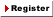Login Member: Email: Password:
 Site Search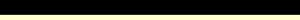Advance Search

Other animationsHow do Optical Fibres work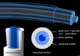Animation product List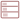Row formatGrid format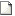List format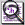Overview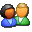(For age - group : Below 16 )

How can two mirrors together can form 2, 5,.. or infinite images, an in-depth explanation of what is actually happening and how a simple equation can govern this. All the cases are taken up and explained using ray diagrams separately. This educational animation is very useful for students who want to understand the concept behind the plain mirror image formation.Product - Animation
 Size/Duration Subscribe Preview / Trailer Size (KB) 330 US\$    0.50 Rs.39.50  Check price in your currency Subscription Days = 30 Watch a preview (opens in separate window) Duration (hr:min:sec) 0:5:0 Add To Cart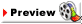Category : Science Type : Animation Animation contains the following explained in detail : How many images will be formed if mirrors are parallel? Ray diagram for explaining the infinite images formation Formation of images in perpendicular mirror system Formula of calculating the images formed in pair of mirrors Explanatory Notes contain: -What is reflection? -Laws of reflection -Why would the secondary image not produce the left-right reversal?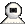Snapshots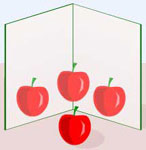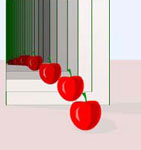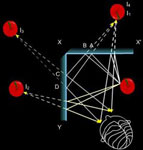Pair of mirrors kept at an right angle to each form 3 images Mirrors kept parallel form infinite images Mirrors kept at right angle to each other form triple images, How ?Details of the animation/ movie /software

The number of images formed for a combination of plane mirrors depends on the angle between the mirrors. If q be the angle between the two mirrors, then the number of images formed is given by,
No. of images, n= 360/q -1

Only the whole number part of n is taken. Further, if n is even then, n = n-1. That is only odd number of images are formed.

For example, if for some value of q, n = 6.2, then n=5.

How do these images form? check out the animation.Related Products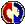Buyers Feedback:Give your comments